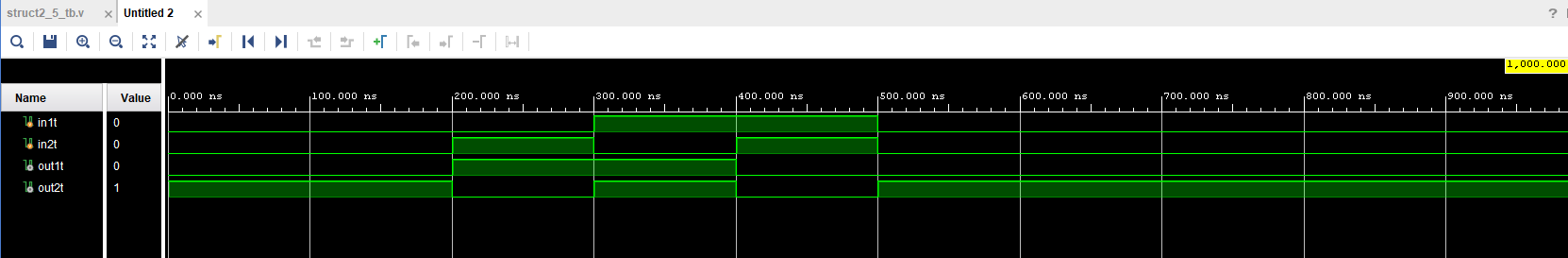CE433 Embedded Devices Spring 2022
Homework 1 - Basics
Name: Ryan Jeanes

Email: rejeanes@fortlewis.edu

Homework 1 - Basics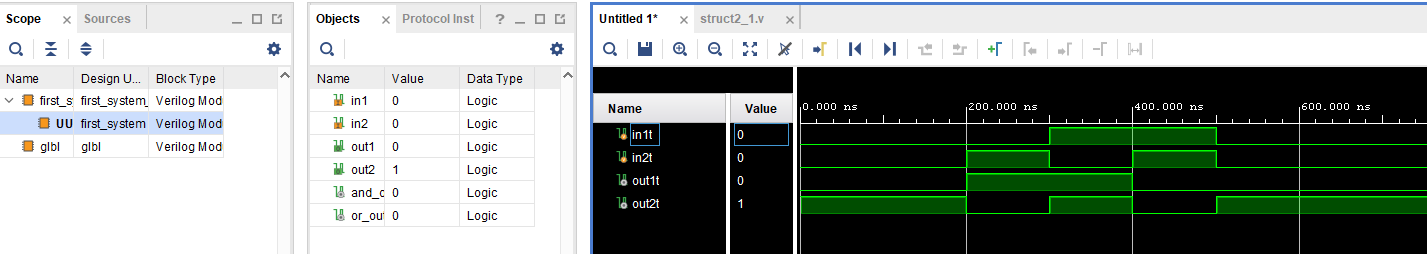Figure 1 - Simulation of section 2.1 structural modeling example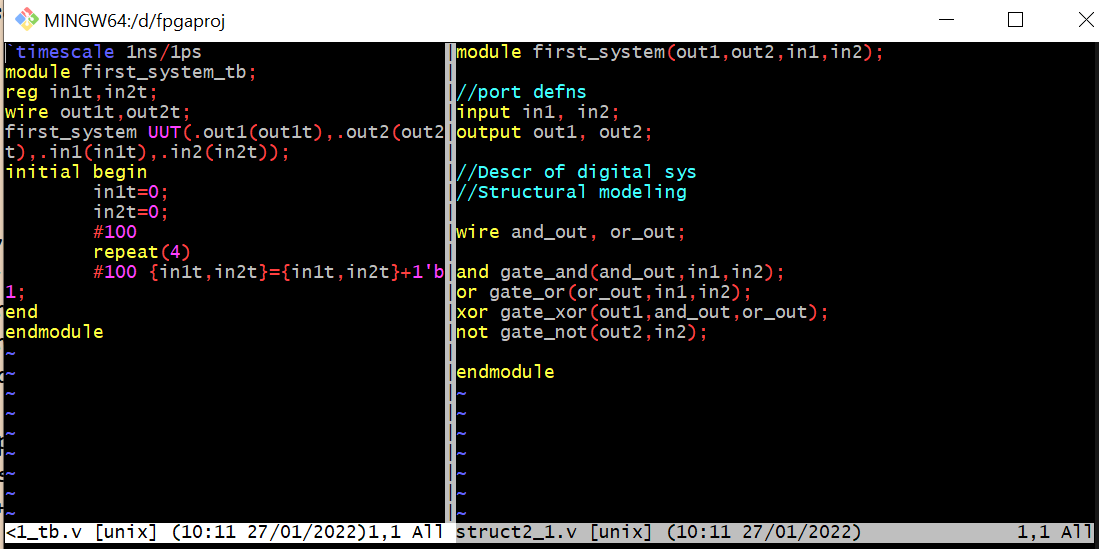Figure 2 - Vim window of section 2.1 structural modeling example.Figure 3 - Simlation of section 2.2 dataflow modeling example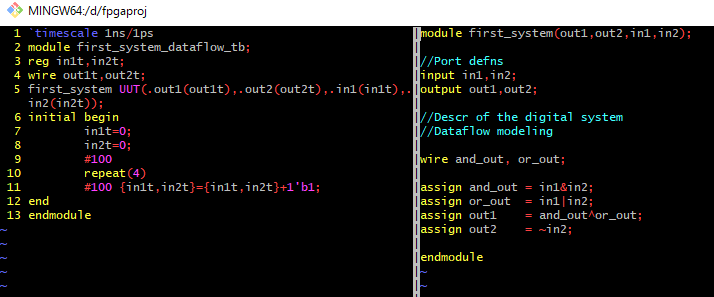Figure 4 - Vim window of section 2.2 dataflow modeling example.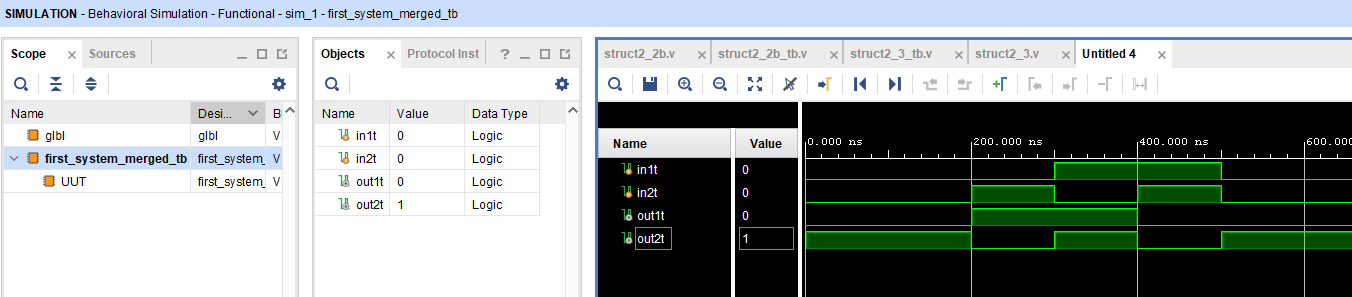Figure 5 - Simulation of section 2.2 merged dataflow modeling example.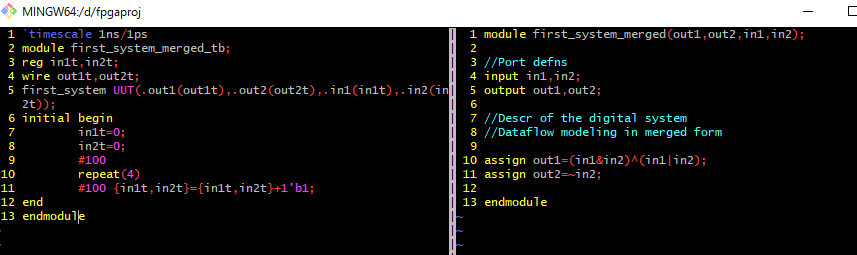Figure 6 - Vim window of section 2.2 merged dataflow modeling example.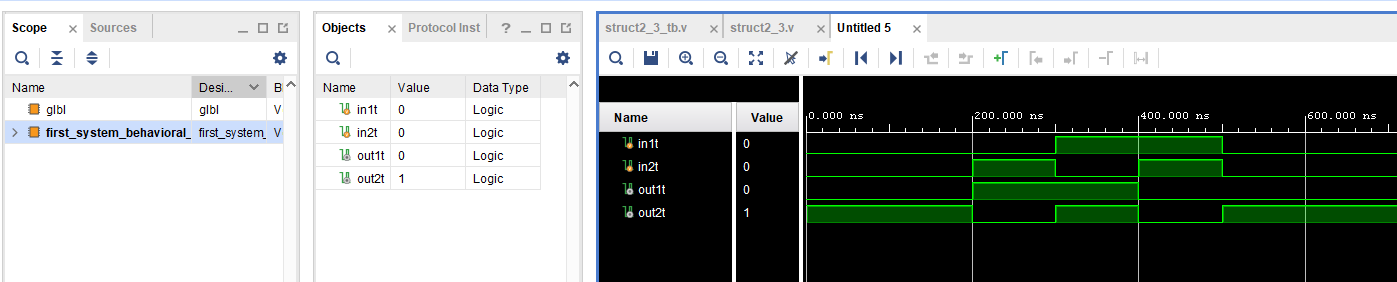Figure 7 - Simulation of section 2.3 example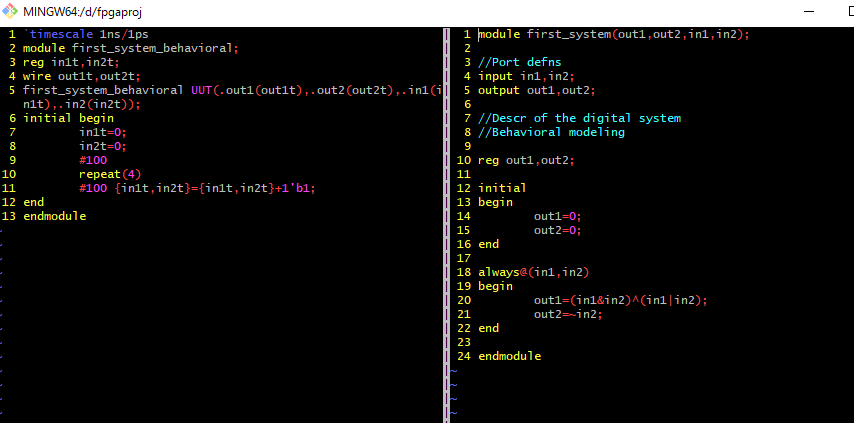Figure 8 - Vim window of section 3.3 example.Figure 9 - Simulation showing the difference of blocking and non-blocking assignment.
Blocking/non-blocking assignments are executed on rising clk edge.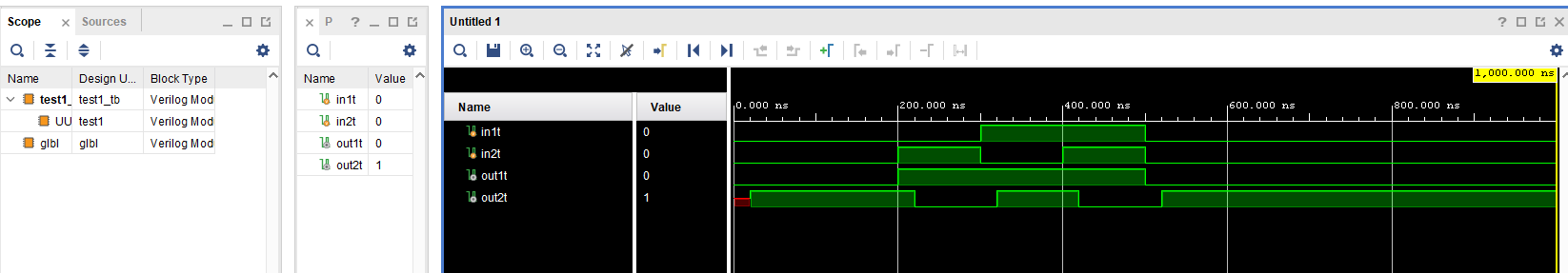Figure 10 - Simulation of section 2.5 example.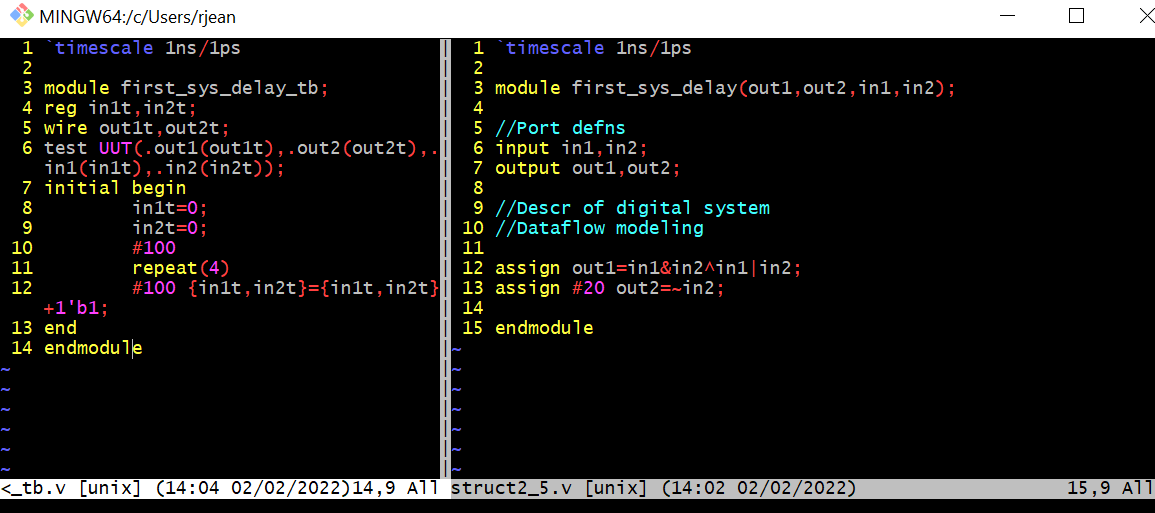Figure 11 - Vim window of section 2.5 example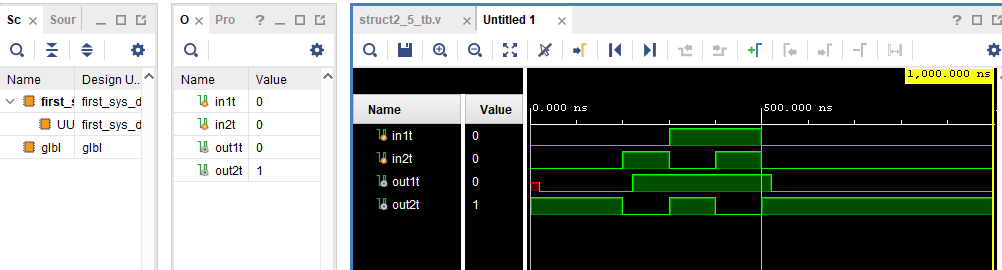Figure 12 - Simulation of section 2.5 example with 20ns delay on line 12 instead of 13. Delay is now shown on out1t.Figure 13 - Vim window of section 2.5 example with 20ns delay on line 12 instead of 13
.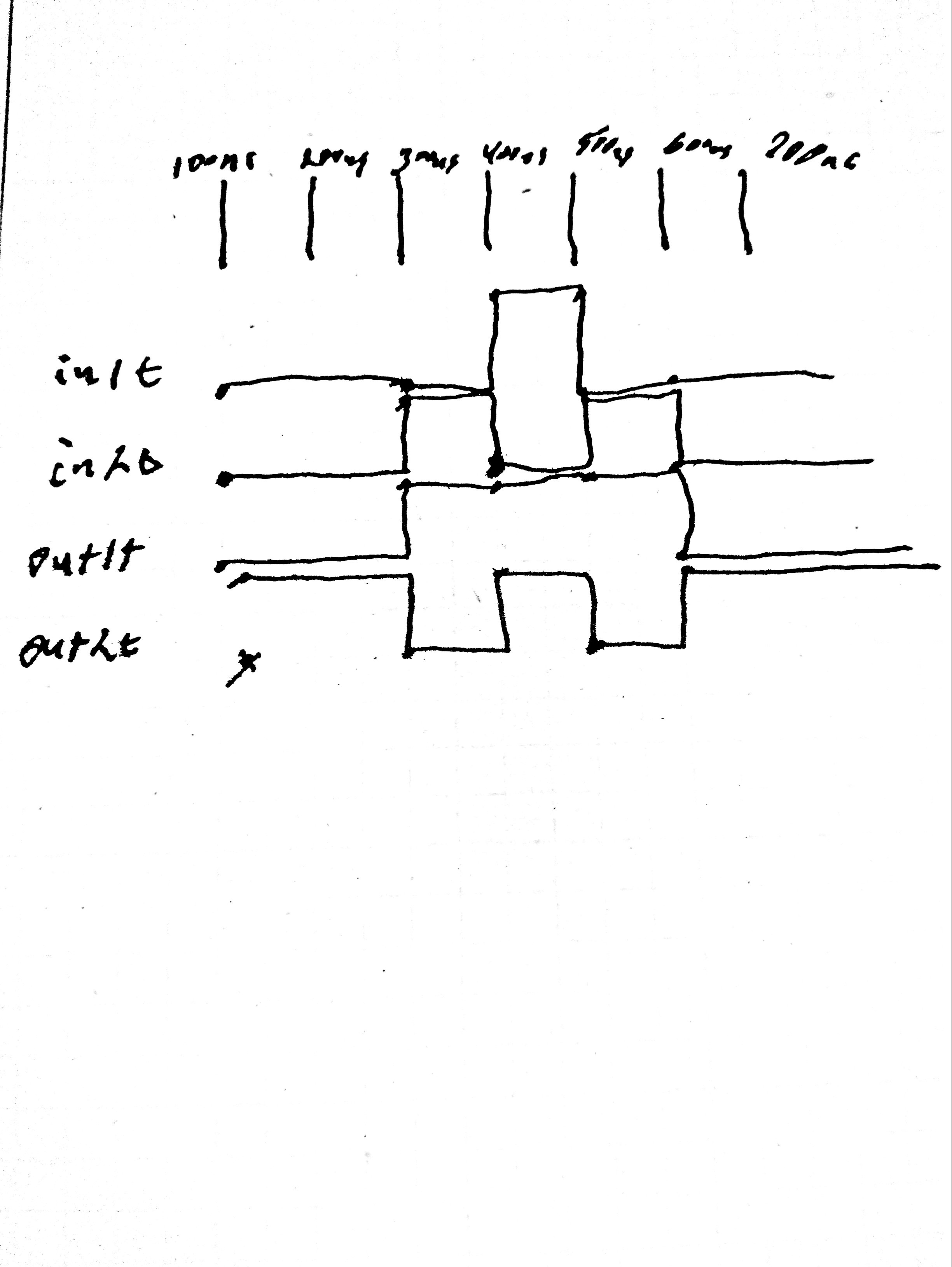Figure 14 - Drawing of given timing diagram.